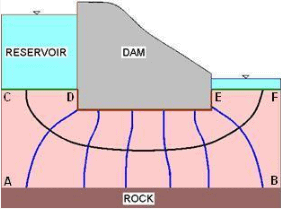Courses

# Procedure for Drawing Flow Nets Civil Engineering (CE) Notes | EduRev

## Civil Engineering (CE) : Procedure for Drawing Flow Nets Civil Engineering (CE) Notes | EduRev

The document Procedure for Drawing Flow Nets Civil Engineering (CE) Notes | EduRev is a part of the Civil Engineering (CE) Course Soil Mechanics.
All you need of Civil Engineering (CE) at this link: Civil Engineering (CE)

At every point (x,z) where there is flow, there will be a value of head h(x,z). In order to represent these values, contours of equal head are drawn.

A flow net is to be drawn by trial and error. For a given set of boundary conditions, the flow net will remain the same even if the direction of flow is reversed. Flow nets are constructed such that the head lost between successive equipotential lines is the same, say Dh. It is useful in visualising the flow in a soil to plot the flow lines, as these are lines that are tangential to the flow at any given point. The steps of construction are:

1. Mark all boundary conditions, and draw the flow cross section to some convenient scale.

2. Draw a coarse net which is consistent with the boundary conditions and which has orthogonal equipotential and flow lines. As it is usually easier to visualise the pattern of flow, start by drawing the flow lines first.

3. Modify the mesh such that it meets the conditions outlined above and the fields between adjacent flow lines and equipotential lines are 'square'.

4. Refine the flow net by repeating step 3.

The most common boundary conditions are:

(a) A submerged permeable soil boundary is an equipotential line. This could have been determined by considering imaginary standpipes placed at the soil boundary, as for every point the water level in the standpipe would be the same as the water level. (Such a boundary is marked as CD and EF in the following figure.)

(b) The boundary between permeable and impermeable soil materials is a flow line (This is marked as AB in the same figure).

(c) Equipotential lines intersecting a phreatic surface do so at equal vertical intervals.

Uses of Flow NetsThe graphical properties of a flow net can be used in obtaining solutions for many seepage problems such as:

1. Estimation of seepage losses from reservoirs: It is possible to use the flow net in the transformed space to calculate the flow underneath the dam.

2. Determination of uplift pressures below dams: From the flow net, the pressure head at any point at the base of the dam can be determined. The uplift pressure distribution along the base can be drawn and then summed up.

3. Checking the possibility of piping beneath dams: At the toe of a dam when the upward exit hydraulic gradient approaches unity, boiling condition can occur leading to erosion in soil and consequent piping. Many dams on soil foundations have failed because of a sudden formation of a piped shaped discharge channel. As the stored water rushes out, the channel widens and catastrophic failure results. This is also often referred to as piping failure.

Offer running on EduRev: Apply code STAYHOME200 to get INR 200 off on our premium plan EduRev Infinity!

## Soil Mechanics

31 videos|48 docs|42 tests

,

,

,

,

,

,

,

,

,

,

,

,

,

,

,

,

,

,

,

,

,

;